Home
Hostname: page-component-56f9d74cfd-s5lbf Total loading time: 0.211 Render date: 2022-06-25T11:11:59.464Z Has data issue: true Feature Flags: { "shouldUseShareProductTool": true, "shouldUseHypothesis": true, "isUnsiloEnabled": true, "useRatesEcommerce": false, "useNewApi": true }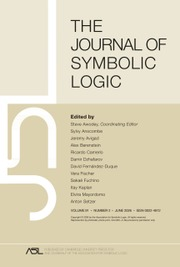The Journal of Symbolic Logic

# On inverse γ-systems and the number of L∞λ-equivalent, non-isomorphic models for λ singular

Published online by Cambridge University Press:  12 March 2014

## Abstract

Suppose λ is a singular cardinal of uncountable cofinality κ. For a model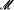of cardinality λ, let No() denote the number of isomorphism types of models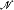of cardinality λ which are L∞λ-equivalent to. In  Shelah considered inverse κ-systems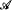of abelian groups and their certain kind of quotient limits Gr()/ Fact(). In particular Shelah proved in [7, Fact 3.10] that for every cardinal Μ there exists an inverse κ-systemsuch thatconsists of abelian groups having cardinality at most Μκ and card(Gr()/ Fact()) = Μ. Later in [8, Theorem 3.3] Shelah showed a strict connection between inverse κ-systems and possible values of No (under the assumption that θκ < λ for every θ < λ): ifis an inverse κ-system of abelian groups having cardinality < λ, then there is a modelsuch that card() = λ and No() = card(Gr()/ Fact()). The following was an immediate consequence (when θκ < λ for every θ < λ): for every nonzero Μ < λ or Μ = λκ there is a model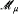, of cardinality λ with No() = Μ. In this paper we show: for every nonzero Μ ≤ λκ there is an inverse κ-systemof abelian groups having cardinality < λ such that card(Gr()/ Fact()) = Μ (under the assumptions 2κ < λ and θ < λ for all θ < λ when Μ > λ), with the obvious new consequence concerning the possible value of No. Specifically, the case No() = λ is possible when θκ > λ for every λ < λ.

## Keywords

Type
Research Article
Information
The Journal of Symbolic Logic , March 2000 , pp. 272 - 284

## Access options

Get access to the full version of this content by using one of the access options below. (Log in options will check for institutional or personal access. Content may require purchase if you do not have access.)

## References

Chang, C. C., Some remarks on the model theory ofinfinitary languages, The syntax and semantics of inflnitary languages (Barwise, J., editor), Lecture Notes in Mathematics, no. 72, Springer-Verlag, Berlin, 1968, pp. 3663.CrossRefGoogle Scholar
Palyutin, E. A., Number of models in L ∞,ω theories, II, Algebra i Logika, vol. 16 (1977), no. 4, pp. 443456, English translation in .Google Scholar
Palyutin, E. A., Number of models intheories, II, Algebra and Logic, vol. 16 (1977), no. 4, pp. 299309.CrossRefGoogle Scholar
Scott, Dana, Logic with denumerably long formulas and finite strings of quantifiers, Theory of models (Proceedings of the 1963 International Symposium, Berkeley) (Addison, J. W., Henkin, Leon, and Tarski, Alfred, editors), North-Holland, Amsterdam, 1965, pp. 329334.Google Scholar
Shelah, Saharon, On the number of nonisomorphic models of cardinality λ L∞,λ-equivalent to a fixed model, Notre Dame Journal of Formed Logic, vol. 22 (1981), no. 1, pp. 510.CrossRefGoogle Scholar
Shelah, Saharon, On the number of nonisomorphic models in L∞,λ when κ is weakly compact, Notre Dame Journal of Formal Logic, vol. 23 (1982), no. 1, pp. 2126.CrossRefGoogle Scholar
Shelah, Saharon, On the possible number no(M) = the number of nonisomorphic models L∞,λ-equivalent to M of power λ, for λ singular, Notre Dame Journal of Formal Logic, vol. 26 (1985), no. 1, pp. 3650.CrossRefGoogle Scholar
Shelah, Saharon, On the no(M) for M of singular power, Around classification theory of models, Lecture Notes in Mathematics, no. 1182, Springer-Verlag, Berlin, 1986, pp. 120134.CrossRefGoogle Scholar
Shelah, Saharon, The number ofpairwisenon-elementarily-embeddable models, this Journal, vol. 54 (1989), no. 4, pp. 14311455.Google Scholar
Shelah, Saharon, Cardinal arithmetic, Oxford Logic Guides, no. 29, The Clarendon Press Oxford University Press, New York, 1994, Oxford Science Publications.Google Scholar
Shelah, Saharon and VÄisÄnen, Pauli, On the number of L∞,λ-equivalent, non-isomorphic models, to appear in Transactions of the American Mathematical Society.Google Scholar

# Save article to Kindle

Note you can select to save to either the @free.kindle.com or @kindle.com variations. ‘@free.kindle.com’ emails are free but can only be saved to your device when it is connected to wi-fi. ‘@kindle.com’ emails can be delivered even when you are not connected to wi-fi, but note that service fees apply.

Find out more about the Kindle Personal Document Service.

On inverse γ-systems and the number of L∞λ-equivalent, non-isomorphic models for λ singular
Available formats
×

# Save article to Dropbox

To save this article to your Dropbox account, please select one or more formats and confirm that you agree to abide by our usage policies. If this is the first time you used this feature, you will be asked to authorise Cambridge Core to connect with your Dropbox account. Find out more about saving content to Dropbox.

On inverse γ-systems and the number of L∞λ-equivalent, non-isomorphic models for λ singular
Available formats
×

# Save article to Google Drive

To save this article to your Google Drive account, please select one or more formats and confirm that you agree to abide by our usage policies. If this is the first time you used this feature, you will be asked to authorise Cambridge Core to connect with your Google Drive account. Find out more about saving content to Google Drive.

On inverse γ-systems and the number of L∞λ-equivalent, non-isomorphic models for λ singular
Available formats
×
×